Courses

# Short Notes - Gravitation Class 9 Notes | EduRev

## Class 9 : Short Notes - Gravitation Class 9 Notes | EduRev

The document Short Notes - Gravitation Class 9 Notes | EduRev is a part of the Class 9 Course Science Class 9.
All you need of Class 9 at this link: Class 9

Facts that Matter

• Universal law of gravitation: Every object in the universe attracts every other object with a force which is proportional to the product of their masses and inversely proportional to the square of the distance between them.

The force is along the line joining the centres of two objects.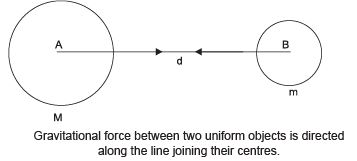Let two objects A and B of masses M and m lie at a distance of d from each other as shown in the figure.
Let F be the force of attraction between two objects. According to the universal law of gravitation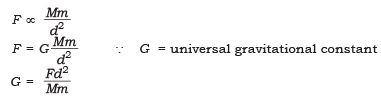• G is called a universal constant because its value does not depend on the nature of intervening medium or temperature or any other physical variable.

S.I. unit of G = Nm2/kg
Value of G = 6.673 × 10−11 Nm2/kg2 (Found by Henry Cavendish)

• Importance of universal law of gravitation
Universal law of gravitation successfully explained several phenomena like :
(i) the force that binds us to the earth.

(ii) the motion of moon around the earth.

(iii) the motion of planets around the Sun.

(iv) the tides due to the moon and the Sun.

• Free fall When an object falls down towards the earth under the gravitational force alone, we say the object is in free fall.
The velocity of a freely falling body changes and is said to be accelerated.
This acceleration is called acceleration due to gravity, denoted by ‘g’. Unit is m/s2.
As F = ma                 (∵ a = g) ...(i)
F = mg                    ...(ii)
and

F = G(Mm/d2)       (from Universal law of gravitation) ...(iii)

From (ii) and (iii)

∴          mg = G(Mm/d2)

∴      g= GM/d2

M = Mass of the earth
d = Distance between the object and the earth
G = Gravitational constant If the object is placed on the earth then
d = R (R = radius of the earth)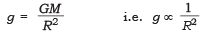...(iv)

Earth is not a sphere, it is flattened at poles.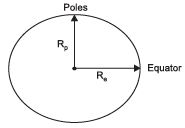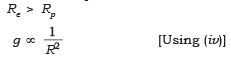∴ The value of ‘g’ is more at poles = (9.9 m/s2) and less at equator = (9.8 m/s2)

Calculation of value of g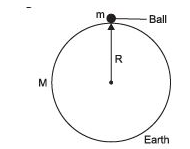g= G(M/R2
G = 6.67 x 10-11 Nm2/kg2

M = 6 × 1024 kg (Mass of the earth)
R = 6.4 × 106 m

On substituting the given values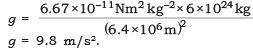Motion of objects under the influence of gravity ‘g’ does not depend on the mass of the body. All objects small, big, heavy, light, hollow or solid fall at same rate.

•  The three equations of motion viz.
(i) v = u + at
(ii) s = ut + (1/2) at2
(iii) v2 – u2 = 2as are true for motion of objects under gravity. For free fall, value of acceleration a = g = 9.8 ms–2.
• If an object is just let fall from a height then in that case u = 0 and a = g = 9.8 m/s2.
• If an object is projected vertically upward with an initial velocity u, then a = – g = – 9.8 ms–2 and the object will go to a maximum height h where its final velocity becomes zero (i.e., v = 0).
• Mass: Mass of an object is the measure of its inertia. It is the matter present in it. It remains the same everywhere in the universe.
• Weight: The force of attraction of the earth on the object is known as the weight of the object. Its S.I. unit is Newton.

W = m × g

The weight of an object can change from one place to the other, from one planet to the other.

Weight of an object on moon is given by the formula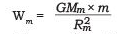Wm = weight of an object on moon
M= Mass of the moon = 7.36 x 1022
Rm = radius of the moon = 1.74 x 106
G = 6.67 x 10-11 Nm2/kg2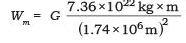Wm = 1.474 × 1010 G × m

and We = 1.474 × 1011 G × m

∴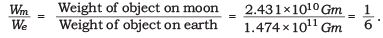∴ Weight of an object on moon = (1/6) th the weight of an object on the earth.

• Thrust: It is the net force applied in a particular direction. Its S.I. unit is N.
• Pressure: It is the force per unit area.

Pressure = thrust / area , S.I unit = N/m2   = Pascal (pa)

• Pressure in fluids
All liquids and gases are fluids, as they can flow.
The pressure exerted by a fluid is transmitted in all direction.
•  Buoyancy
The upward force exerted by water (fluid) on the body is known as upthrust or buoyancy.

The magnitude of the buoyant force depends on the density of the fluid.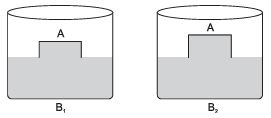A − Wooden block
B1 − Beaker with tap water
B2 − Beaker with salty water
The buoyant force exerted in B2 is more as compared to B1. It is because the density of B2 water is more.

The object may float, sink or remain half submerged and half above water, the density of the fluid and object decides this.
– If the density of object is less than the fluid, then it will float on the liquid.
– If the density of object is more than the fluid, then it will sink in the liquid.
– If the density of object is same as the density of fluid, then it will half float and half sink.

•  Archimedes’ principle: When a body is immersed fully or partially in a fluid, it experiences an upward force that is equal to the weight of the fluid displaced by it.
• Its applications are:

(i) Used in designing ships and submarines,
(ii) In lactometers to find purity of milk.
(iii) In hydrometer to determine density of liquid.
Relative density = density of substance / density of water

It has no unit.

Offer running on EduRev: Apply code STAYHOME200 to get INR 200 off on our premium plan EduRev Infinity!

## Science Class 9

143 videos|224 docs|135 tests

,

,

,

,

,

,

,

,

,

,

,

,

,

,

,

,

,

,

,

,

,

;